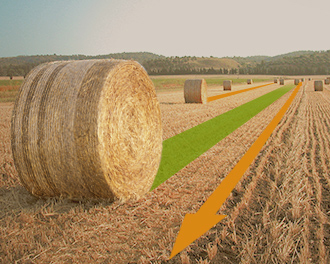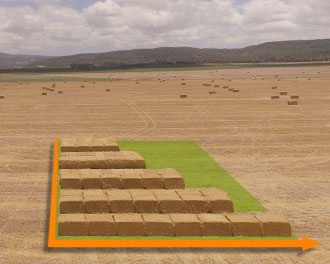## Film for square bales Example 2

There are many varying sizes of square bale wrapped, making calculating the number of bales per roll complex. The quantity of bales wrapped, of any dimension, from one roll of film is dependent on a number of important factors.

• THE BALE SIZE

• THE % STRETCH APPLIED TO THE FILM

• THE NUMBER OF TURNS GIVEN TO THE BALE

By using the following formula, a calculation of the approximate number of square bales possible from one roll of film can be made

 EXAMPLE – 1.2m x .60m x .80m bale – 4 layers of film A Add the sum of the bale’s four largest dimensions = 4m     (2 x 1.2m + 2 x .80m) B Multiply by 75 (75cm Film width) C Multiply by 0.0025 (25 micron Film thickness) D Multiply by 0.0933 (Film Density) E Divide by 1.7 (Film length plus 70% stretch) F Multiply by 16 (Number of turns of the bale for 4 film layers) = 0.658kg OF FILM PER BALE G Divide into weight of film on roll (not including core) (approximately 26kg per 750mm x 1500m roll) 26kg (total film weight / roll) ÷ 0.658kg (film weight / bale) = 39 BALES PER ROLL

 EXAMPLE – 1.2m x .60m x .80m bale – 6 layers of film A Add the sum of the bale’s four largest dimensions = 4m     (2 x 1.2m + 2 x .80m) B Multiply by 75 (75cm Film width) C Multiply by 0.0025 (25 micron Film thickness) D Multiply by 0.0933 (Film Density) E Divide by 1.7 (Film length plus 70% stretch) F Multiply by 24 (Number of turns of the bale for 6 film layers) = 0.988kg OF FILM PER BALE G Divide into weight of film on roll (not including core) (approximately 26kg per 750mm x 1500m roll) 26kg (total film weight / roll) ÷ 0.988kg (film weight / bale) = 26 BALES PER ROLL
Upptäck mer
NätkalkylatorBeställ rätt antal Räkna Tama AssistBred värld av kunskap Enter GarnväljareVälj rätt garn Enter GarnkalkylatorBeställ rätt antal Räkna
Upp